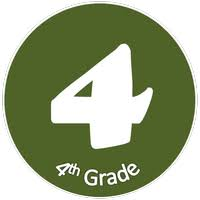Price \$35/Session
Duration 1 Session
Credit Week

Course Description

Course Objective: The students will receive instruction in and demonstrate the ability to perform basic mathematical functions and problem-solving in the areas of addition, subtraction, multiplication, and division of whole numbers, decimals, fractions, and geometry.

Textbook: Math 4

Materials:

• Student textbook
• Practice and enrichment worksheets
• Manipulative materials
• Calculators

Time Allotment:

• 45 minutes per day, 2 days per week

Course Content:

• Data and Graphs
• Place Value
• Adding and Subtracting Whole Numbers, Money, and Decimals
• Multiplication of Whole Numbers
• Division of Whole Numbers
• Fractions
• Geometry
• Measurement
• Time
• Decimals
• Problem Solving

Areas to be evaluated:

• Class participation
• Homework assignments
• Computation time tests
• Tests
• Quizzes